• FAQ
• Contact/
/
/
31When there increasing returns to scale, average costs must
Not my Question
Flag Content

# Question : 31When there increasing returns to scale, average costs must : 1753217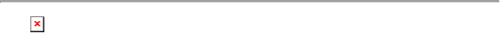31When there are increasing returns to scale, average costs must be:

falling.

rising.

constant.

falling, then rising.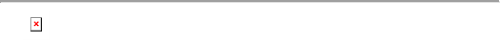32Whenever a firm's marginal costs are less than its average costs, its average costs must be:

falling.

rising.

constant.

falling, then rising.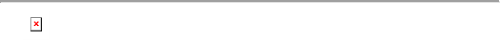33Increasing returns to scale and declining average costs result from all of the following except:

focusing on a single product line and specializing.

exporting goods to other countries.

selling more in their home market

hiring more workers at the existing plant.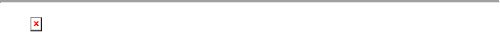34A firm's average costs will be falling whenever its:

marginal costs are positive.

marginal costs are negative.

marginal costs are less than average costs.

marginal costs are less than fixed costs.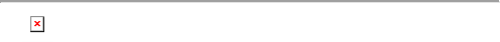35Firm X's total fixed costs are \$1,000. Its total variable costs of producing 100 units are \$2,000, and its total variable costs of producing 200 units are \$4,000. Firm X's average costs experiences which of the following as it increases output from 100 to 200 units?

Average costs increase.

Average costs decrease.

Average costs remain constant.

Average costs increase slightly.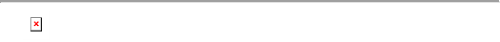36Consider the following cost information for a monopolist: MR =

\$15, MC = \$23, and the quantity is 9. Which of the following statements is correct?

The monopolist should produce and sell 9 units of output.

The monopolist should increase production of output.

We need more information to decide if the firm needs to produce.

The monopolist should not produce this output because MR <MC.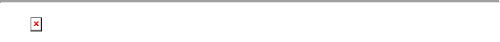37At its current production level, a monopolist's marginal revenue is \$20 and its marginal cost is \$10. Which of the following statements is correct?

The monopolist should produce and sell more output.

The monopolist should produce and sell less output.

The monopolist is maximizing its profits at its current level of output.

More information is required to decide if the firm needs to change its production.38A monopolistic competitor has fixed costs of \$100 and marginal costs of \$10 per unit. What is its average cost of producing 100

units?

\$10

\$11

\$1,100

\$2,00039A monopolistic competitor has fixed costs of \$100 and marginal costs of \$10 per unit. What is its marginal revenue at its

equilibrium price and quantity?

\$10

\$11

\$1,100

\$2,00040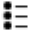If a firm has a total cost of \$150 and a variable cost of \$100 for producing 5 units of output, then the fixed cost is:

\$35.

\$50.

\$250.

\$100.

## Solution 5 (1 Ratings )

Solved
Business Management 1 Year Ago 37 Views